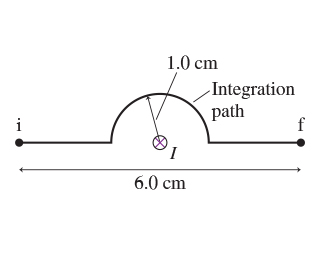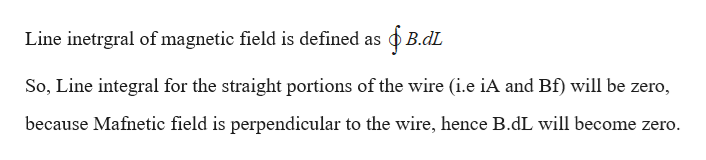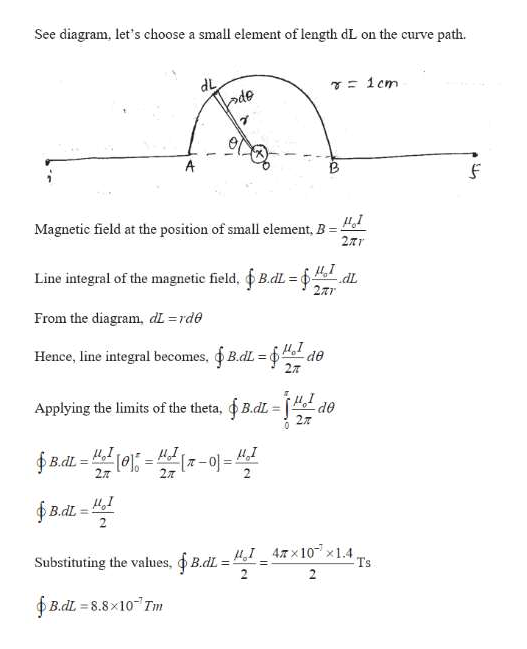Question
6 views
What is the line integral of B  between points i and f in the figure? (Figure 1) Assume that I = 1.4 A .help_outlineImage Transcriptionclose1.0 cm Integration path f i I 6.0 cm fullscreen
check_circle

Step 1

Given:

Current flowing in the wire = 1.4 A

Step 2

Calculating the line integral of B for straight wire portions:help_outlineImage TranscriptioncloseLine inetrgral of magnetic field is defined as B.dL So, Line integral for the straight portions of the wire (i.e iA and Bf) will be zero, because Mafnetic field is perpendicular to the wire, hence B.dL will become zero. fullscreen
Step 3

Line integral for the curve portion ...help_outlineImage TranscriptioncloseSee diagram, let's choose a small element of length dL on the curve path T1cm ode A Magnetic field at the position of small element, B 2Tr Line integral of the magnetic field, B.dL dL 2r From the diagram, dL=rde de Hence, line integral becomes, B.dL 2T Applying the limits of the theta, B.dL d 0 I'n 2 B.dL 7-0 2T 2T BdL 2 4,I47x10x1.4, Ts Substituting the values,B.dL 2 B.dL 8.8x10 Tm fullscreen

### Want to see the full answer?

See Solution

#### Want to see this answer and more?

Solutions are written by subject experts who are available 24/7. Questions are typically answered within 1 hour.*

See Solution
*Response times may vary by subject and question.
Tagged in

### Magnetic Effects of Current